Electron. J. Differential Equations, Vol. 2019 (2019), No. 32, pp. 1-29.

### Bifurcation from the first eigenvalue of the p-Laplacian with nonlinear boundary condition Mabel Cuesta, Liamidi A. Leadi, Pascaline Nshimirimana

Abstract:
We consider the problem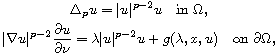where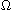is a bounded domain of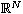with smooth boundary,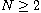, and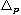denotes the p-Laplacian operator. We give sufficient conditions for the existence of continua of solutions bifurcating from both zero and infinity at the principal eigenvalue of p-Laplacian with nonlinear boundary conditions. We also prove that those continua split on two, one containing strictly positive and the other containing strictly negative solutions. As an application we deduce results on anti-maximum and maximum principles for the p-Laplacian operator with nonlinear boundary conditions.

Submitted July 19, 2018. Published February 21, 2019.
Math Subject Classifications: 35J92, 35J65, 35J60, 35B32, 35B50.
Key Words: Bifurcation theory; topological degree;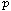-Laplacian; elliptic problem; nonlinear boundary condition; maximum and anti-maximum principles.

Show me the PDF file (400 KB), TEX file for this article.Mabel Cuesta Laboratoire de Mathématiques Pures et Appliquées Université du Littoral Côte d'Opale (ULCO) 50, rue F. Buisson, BP: 699 62228 Calais, France email: cuesta-l@univ-littoral.fr Liamidi Leadi Département de Mathématiques, Faculté des Sciences et Techniques Institut de Mathématiques et de Sciences Physiques (IMSP) Université d'Abomey-Calavi (UAC) 01 BP 613, Porto-Novo, Benin email: leadiare@imsp-uac.org, leadiare@yahoo.com Pascaline Nshimirimana Institut de Mathématiques et de Sciences Physiques (IMSP) Université d'Abomey-Calavi (UAC) 01 BP 613, Porto-Novo, Benin email: pascaline.nshimirimana@imsp-uac.org, nshimirimanapascaline@yahoo.fr